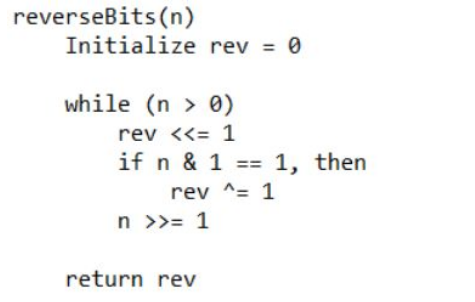# Reverse Bits

Problem Statement: The problem is to reverse the bits of n and print the number obtained after reversing the bits.

Examples:

```Example 1:
Input: 6
Output: 3
Explanation:
(6)10 = (110)2.
After reversing the bits we get:
(011)2 = (3)10.

Example 2:
Input: 11
Output: 13
Explanation:
(11)10 = (1011)2.
After reversing the bits we get:
(1101)2 = (13)10.
```

### Solution

Disclaimer: Don’t jump directly to the solution, try it out yourself first.

### Solution 1:

In example 1, The binary of 6 is 110 if we reverse 110 then we will get 011 which is the binary of 3. So, all we have to do is to convert the decimal number(base 10) into binary(base 2) and then reverse the binary number and then convert it into decimal.One by one bit in the binary representation of n is being obtained with the help of bitwise right shift operation and they are being accumulated in rev with the help of bitwise left shift operation.

Right Shift :

Takes two numbers, right shifts the bits of the first operand, and the second operand decides the number of places to shift.

```eg: let's take N=32; which is 100000 in Binary Form.
If “N is right-shifted by 2” i.e N=N>>2 then N will become N=N/(2^2). Thus, N=32/(2^2)=8 which can be written as 1000.
```

Left Shift :

Takes two numbers, the left shifts the bits of the first operand, and the second operand decides the number of places to shift.

```eg: let's take N=22; which is 00010110 in Binary Form.
If “N is left-shifted by 2” i.e N=N<<2 then N will become N=N*(2^2). Thus, N=22*(2^2)=88 which can  be written as 01011000.```

Code:

## C++ Code

``````#include<iostream>
using namespace std;

int reverseBits(int n)
{
int rev = 0;
while (n > 0) {
rev <<= 1;

if (n & 1 == 1)
rev ^= 1;
n >>= 1;
}

return rev;
}

int main()
{
unsigned int n = 6;
cout<< reverseBits(n);
return 0;
}
``````

Output: 3

Time Complexity: 0(n)

Space Complexity: 0(1)

## Java Code

``````public class ABC{
public static int revBits(int n){
int rev_n = 0;
while (n > 0){
rev_n <<= 1;
if ((int) (n & 1) == 1){
rev_n ^= 1;
}
n >>= 1;
}
return rev_n;
}
public static void main(String[] args){
int n =6 ;

System.out.println(revBits(n));
}
}``````

Output: 3

Time Complexity: 0(n)

Space Complexity: 0(1)

Special thanks to AYUSH KUMAR for contributing to this article on takeUforward. If you also wish to share your knowledge with the takeUforward fam, please check out this articleIf you want to suggest any improvement/correction in this article please mail us at [email protected]